Question

# Objective: Add the hexadecimal value 0A3F from a memory location to the hexadecimal value 0FF2 in...

Objective: Add the hexadecimal value 0A3F from a memory location to the hexadecimal value 0FF2 in a register and store the result in the memory. Subtract one from the result, then move the result to both another register and another memory location.

1. Draw a flowchart to break down the steps and storage locations for this program.

Program and flowchart is attached herewith.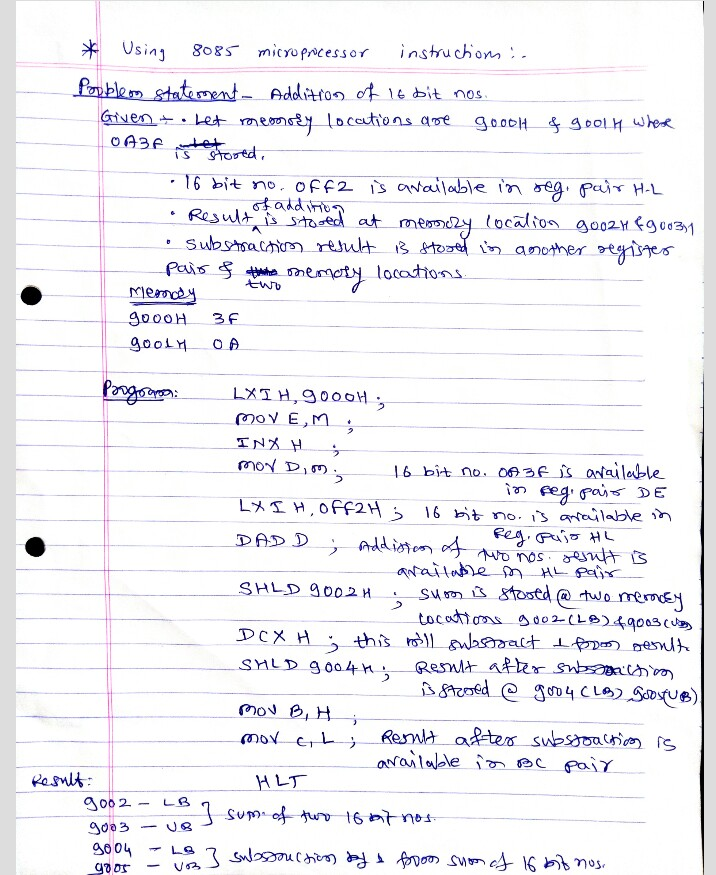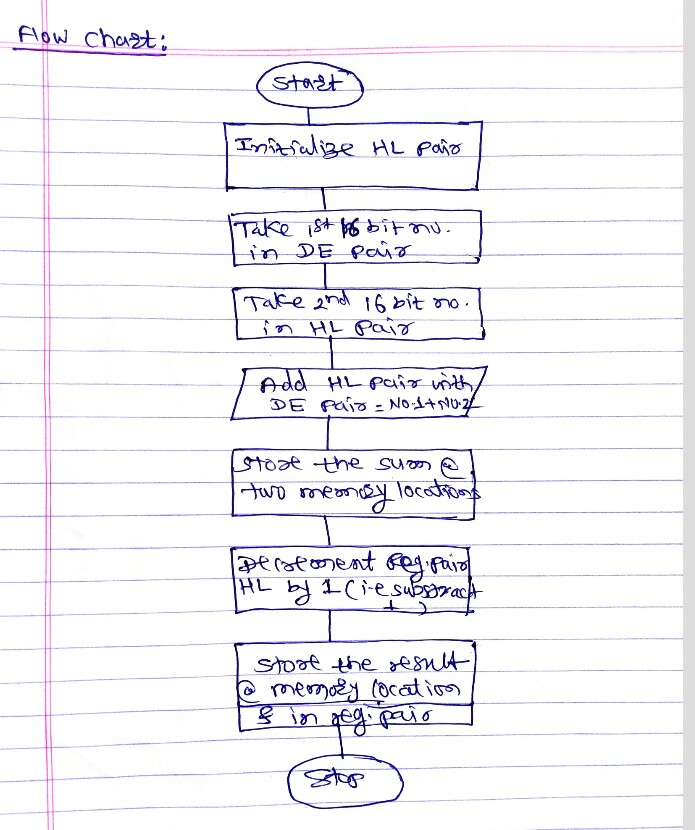#### Earn Coins

Coins can be redeemed for fabulous gifts.

Similar Homework Help Questions
• ### Write a program in assembly language that loads register R2 with the word in memory location...

Write a program in assembly language that loads register R2 with the word in memory location which is 10 bytes above the address in R0; and loads register R3 with the word in memory location which is 10 bytes below the address in R1. Your program must compare the two numbers in R2 and R3. If number in R2 is less than or equal to the number in R3 it must add the two numbers and save the result in...

• ### A list of numbers (in hexa) is stored in the memory location range of (0000 –...

A list of numbers (in hexa) is stored in the memory location range of (0000 – 00FF), we need to subtract \$04 from each number of the list. Solve this problem using assembly language, use the Indexed addressing in writing of your program. Store the result of subtracting each number in the same memory location.

• ### The AVR provides a rich instruction set to support high-level languages. The AVR address- ing modes...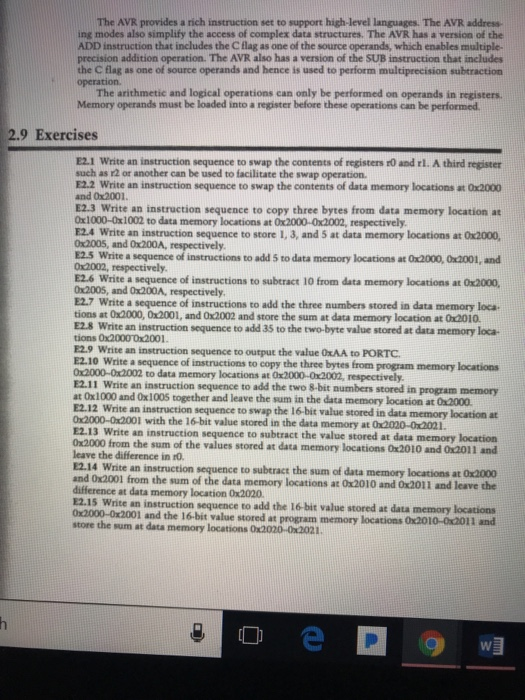The AVR provides a rich instruction set to support high-level languages. The AVR address- ing modes also simplify the access of complex data structures. The AVR has a version of the ADD instruction that includes the C flag as one of the source operands, which enables multiple- precision addition operation. The AVR also has a version of the SUB instruction that includes the C flag as one of source operands and hence is used to perform multiprecision subtraction operation. The...

• ### Problem: Write the instructions to add the value \$16 and SCD. Place the result in register...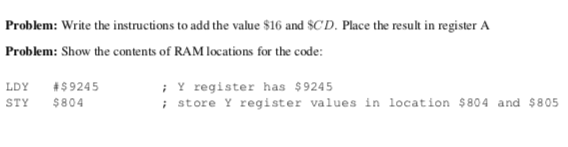Problem: Write the instructions to add the value \$16 and SCD. Place the result in register A Problem: Show the contents of RAM locations for the code: LDY #\$9245 STY \$804 Y register has \$9245 store Y register values in location \$804 and \$805

• ### Write a program using PIC24 instructions yo add three 8-bit numbers stored in the successive memory...

Write a program using PIC24 instructions yo add three 8-bit numbers stored in the successive memory locations starting from 0x0800. Store the result in the memory lpcation 0x0855.

• ### Instruction set architecture R: register X, Y, Opl, Op2: Operand Quantity: constant value EA: Effective memory address Opcode Operation Name MOV X, Y XCH Opl, Op2 ADD X, Y SUB X,Y SAL Op, Quantity...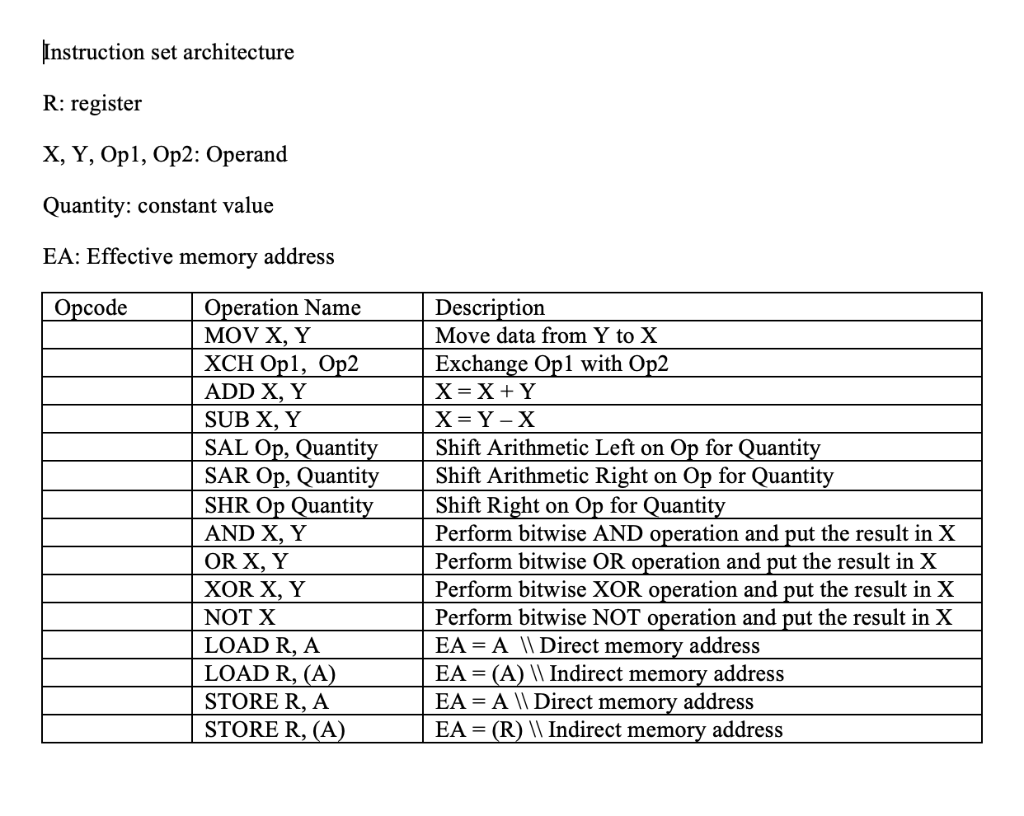Instruction set architecture R: register X, Y, Opl, Op2: Operand Quantity: constant value EA: Effective memory address Opcode Operation Name MOV X, Y XCH Opl, Op2 ADD X, Y SUB X,Y SAL Op, Quantity SAR Op, Quantity Shift Arithmetic Right on Op for Quantity SHR Op Quanti AND X, Y OR X, Y XOR X, Y NOT X LOADR, A LOAD R, (A STORE R, A STORE R, (A Description Move data from Y to X Exchange Op1 with Op2...

• ### ) Identify the hexadecimal value moved to the destination by each of the following instruction below...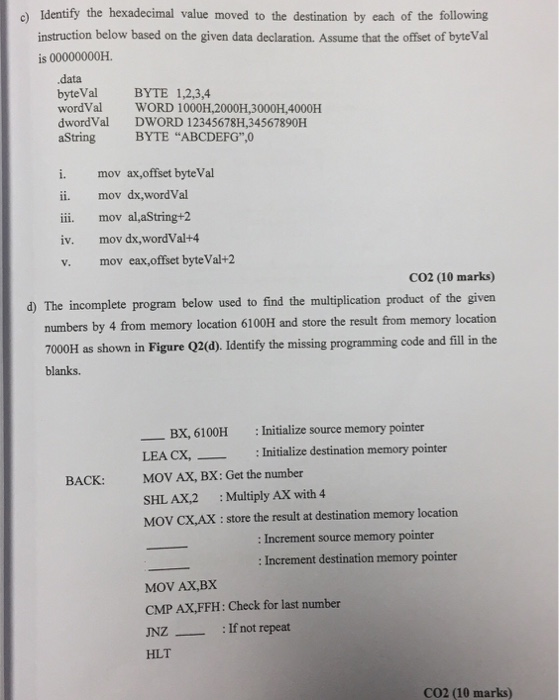) Identify the hexadecimal value moved to the destination by each of the following instruction below based on the given data declaration. Assume that the offset of byteVal is 00000000H data byteVal wordVal dwordVal aString BYTE 1,2,3,4 WORD 1000H,2000H,3000H,4000H DWORD 12345678H,34567890H BYTE "ABCDEFG",0 ax,offset byteVal i. mov dx,wordVal i. mov al,aString+2 i11. mov dx,wordVal+4 iv. mov mov eax,offset byteVal+2 V. CO2 (10 marks) d) The incomplete program below used to find the multiplication product of the given numbers by 4...

• ### 1. Translate the following tasks into a single ARM instruction: a. Add 32 times of the...

1. Translate the following tasks into a single ARM instruction: a. Add 32 times of the content of registers r0 and the content of r1 only if N is clear. Store the result in register r2 b. Subtract the content of register r0 from 0x990 and put the results in register r3 only if C is set and Z is clear. c. Clear the 2nd least significant byte of the content of register r1, i.e., store (00000000)2 in it, and...

• ### The hypothetical machine of Figure 3.4 also has two I/O instructions:0011 = Load AC from I/O0111...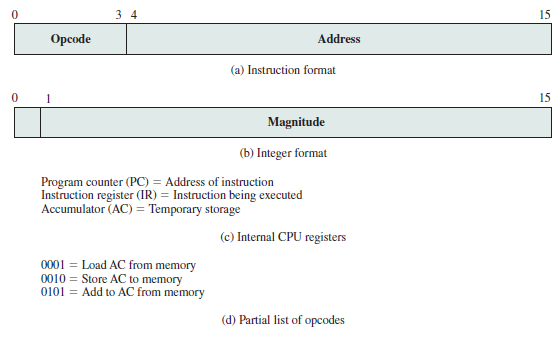The hypothetical machine of Figure 3.4 also has two I/O instructions:0011 = Load AC from I/O0111 = Store AC to I/OIn these cases, the 12-bit address identifies a particular I/O device. Show the program execution (using the format of Figure 3.5) for the following program:1. Load AC from device 5.2.  Add contents of memory location 940.3.  Store AC to device 6.Assume that the next value retrieved fromdevice 5 is 3 and that location 940 contains a value of 2.Example of Program...

• ### A microprocessor has a decrement memory direct instruction, which subtracts 1 from the value in a...

A microprocessor has a decrement memory direct instruction, which subtracts 1 from the value in a memory location. The instruction has five stages: fetch opcode (two bus clock cycles), fetch operand address (five cycles), fetch operand (five cycles), subtract 1 from operand (four cycles), and store operand (two cycles). a. By what amount (in percent) will the duration of the instruction increase if we have to insert one bus wait state in each memory read and memory write operation? b....